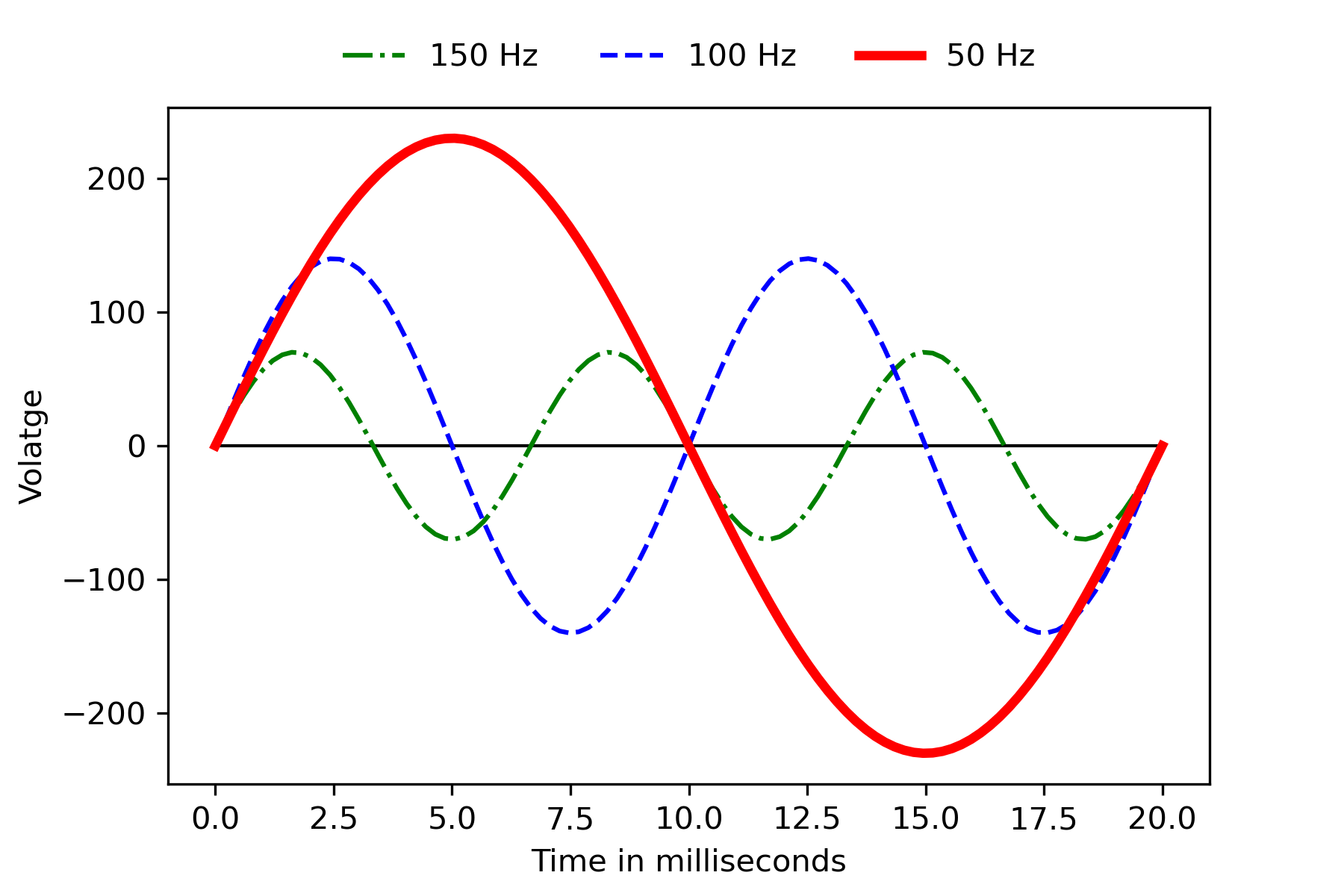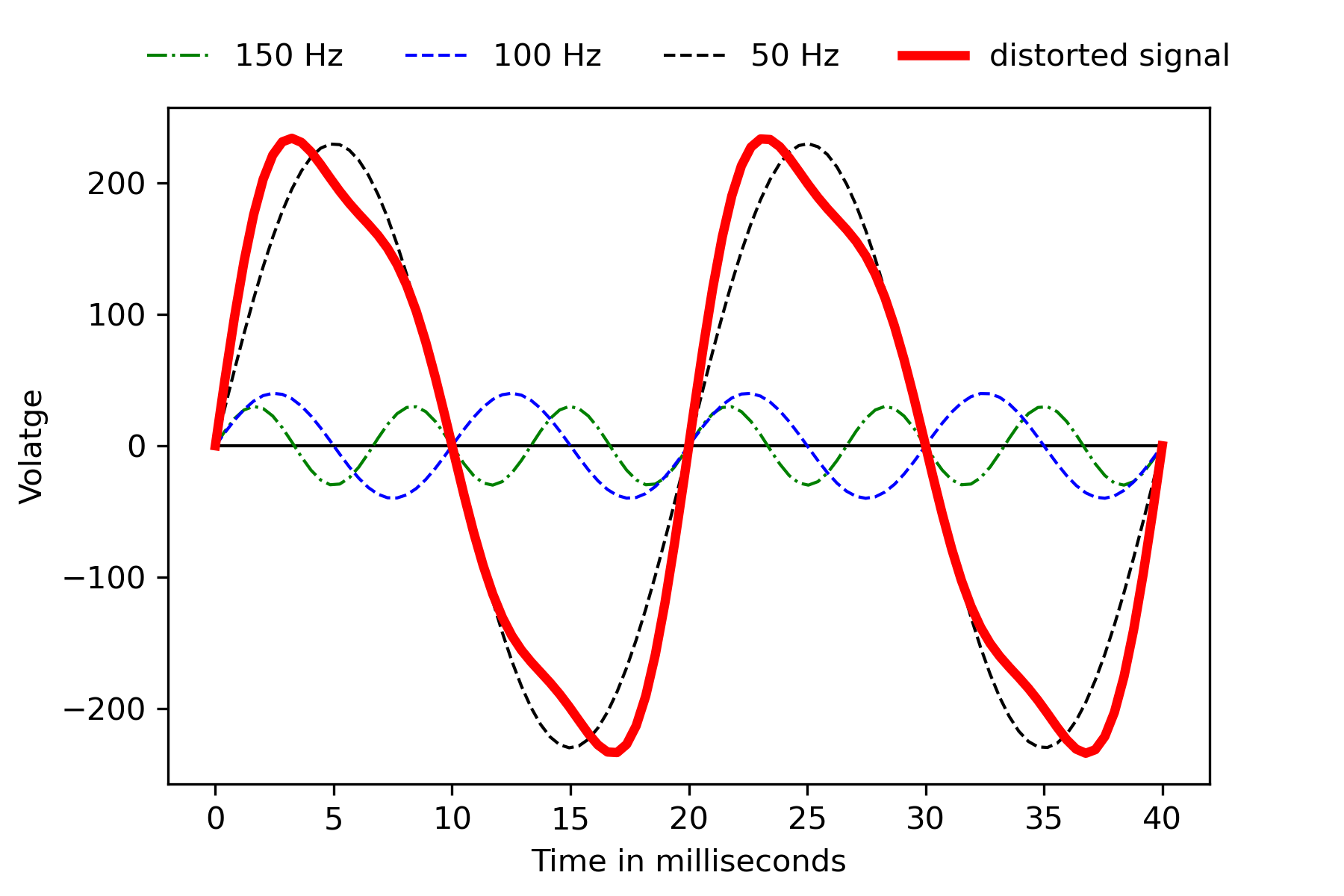29 Nov
29Nov

## Harmonics in wind power plants - Part 1

On the path to a climate-neutral future the current energy transition is playing an indispensable role. However, with the advent of electric cars and the increasing digitalization of our society, we face a problem: Where do we get the necessary electrical energy (and, above all, in a sustainable way)? Wind energy is a key part of this solution. Wind turbines, whether onshore or offshore, have produced just about 740 GW of power in 2020. This represents a contribution of about 6% of the global electricity production, and the share is growing. But with the use of renewable energies, our power grid is also facing new problems. In the case of wind turbines harmonics in particular are a restraining factor. That is exactly why we want to provide you with two blogposts explaining why harmonics are a problem and how to counteract them. This first part focuses on the definition and challenges posed by harmonics.

### The definition of harmonics

Our power grid mainly operates with alternating quantities (alternating voltage and alternating current). As the name suggests, alternating current changes its direction permanently and alternating voltage changes its polarity accordingly. In the Central European power grid voltage and current change 50 times per second. Ideally, this happens with a sinusoidal pattern (Figure 1). Therefore, our power grid also has a frequency of 50 Hz. In the USA, on the other hand, the grid frequency is 60 Hz. Harmonic frequencies (short: harmonics or higher modes) are multiples of one of these fundamental frequencies. For example, if a voltage signal in Europe has a frequency of 100 Hz (2×50 Hz), this is a 2nd order harmonic. A frequency of 150 Hz (3×50 Hz) is a 3rd order harmonic, and so on. In addition to the fundamental frequency of 50 Hz, Figure 1 also shows the second and third order harmonics.Figure 1: Second and third order harmonics in a voltage signal

Now, the question naturally arises: Can there be frequencies which are not multiples of the fundamental frequency? And indeed, this is the case. We call such frequencies interharmonics. Examples are frequencies of 76 Hz or 114 Hz. The same considerations apply to them as to harmonics.

### What influence do harmonics have?

But why are higher modes such a problem? It is because our power grid and the devices connected to it rely on being supplied with a perfectly sinusoidal signal at the correct frequency. But that is exactly what harmonics prevent. The more harmonics present in the signal, the further it deviates from the ideal sinusoidal curve. Let us consider an example: The fundamental frequency of a voltage signal is 50 Hz. Now we add two higher modes with 100 Hz and 150 Hz. If we add all three voltage signals together, the result is no longer sinusoidal (Figure 2).Figure 2: Harmonic distortion of a pure sine wave

But what does this mean for us in practice? As mentioned, the majority of components in our power grid are optimized for the fundamental frequency. Harmonics can therefore lead to heat generation and vibrations in transformers, cables and all other equipment. Taking wind turbines as an example, this reduces the service life of (mainly electronic) components. Malfunctions in a wide variety of areas (e.g. in safety systems) can also happen. The reason for this is that many devices are calibrated to an exact zero crossing of the sine curve. If the zero crossing deviates from the ideal due to harmonics, malfunctions can occur. In fact, it is possible that up to 20% of the produced power is in the form of harmonics. This significantly reduces the efficiency of a wind turbine. It becomes especially noticeable when harmonics coincide with a so-called resonant frequency. In this case, the corresponding harmonic frequency is (unintentionally) amplified by the wind power plant. The resonance frequencies are not always known. They depend, amongst other factors, on the design of the wind turbine and even on the wind speed. Finally, the stability of our power supply also decreases as harmonics increase. If the frequency of the entire power grid drops only from 50 Hz to 47.5 Hz, large-scale grid failures will occur. This and the previously mentioned factors are reasons enough to avoid harmonics in practice. How to do this will be discussed in our blog post next week.